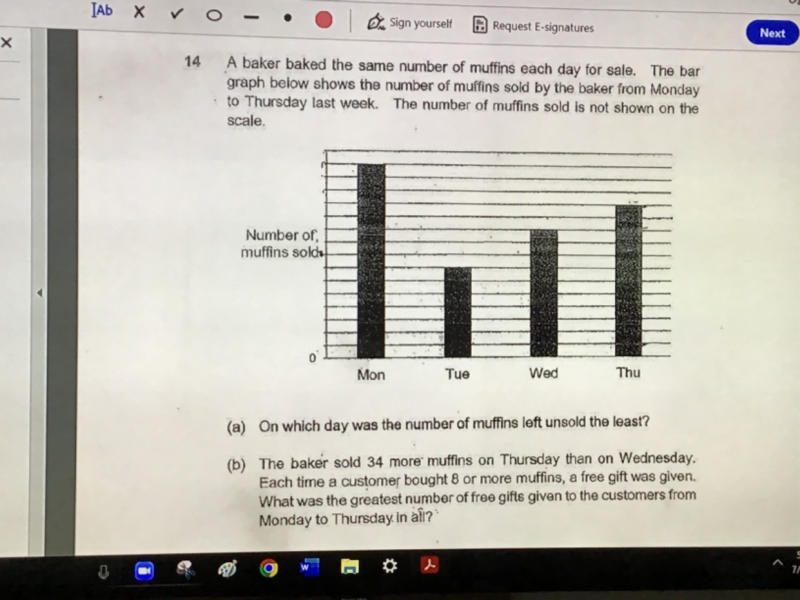# QuestionIs the answer for (b) 91 or 93? Thank you!

(b) 2u -> 34 => u = 17

M -> 15u   = 15 x 17 = 255     255/8 = 31 r 7

Tu -> 7u    = 7 x 17  = 119       119/8 = 14 r 7

W -> 10u    = 10 x 17 = 170     170/8 = 21 r 2

Th -> 12u   = 12 x 17 = 204      204/8 = 25 r 4

Hence greatest number of free gifts given to customers from Mon to Thu

= 31+14+21+25 = 91

You have to calculate the free gifts promotion day by day as the muffins are sold daily and given out per transaction.

0 Replies 1 Like ✔Accepted Answer